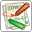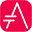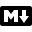# Network diagram (nwdiag)nwdiag has been created by Takeshi Komiya and allows to quickly draw network diagrams. So we thank him for his creation!

Since the syntax is clear and simple, this has been integrated within PlantUML. We reuse here the examples that Takeshi has documented.

## Simple diagram```@startuml nwdiag { network dmz { address = "210.x.x.x/24" web01 [address = "210.x.x.1"]; web02 [address = "210.x.x.2"]; } network internal { address = "172.x.x.x/24"; web01 [address = "172.x.x.1"]; web02 [address = "172.x.x.2"]; db01; db02; } } @enduml ```## Define multiple addresses```@startuml nwdiag { network dmz { address = "210.x.x.x/24" // set multiple addresses (using comma) web01 [address = "210.x.x.1, 210.x.x.20"]; web02 [address = "210.x.x.2"]; } network internal { address = "172.x.x.x/24"; web01 [address = "172.x.x.1"]; web02 [address = "172.x.x.2"]; db01; db02; } } @enduml ```## Grouping nodes### Define group inside network definitions

```@startuml nwdiag { network Sample_front { address = "192.168.10.0/24"; // define group group web { web01 [address = ".1"]; web02 [address = ".2"]; } } network Sample_back { address = "192.168.20.0/24"; web01 [address = ".1"]; web02 [address = ".2"]; db01 [address = ".101"]; db02 [address = ".102"]; // define network using defined nodes group db { db01; db02; } } } @enduml ```### Define group at outside network definitions

```@startuml nwdiag { // define group at outside network definitions group { color = "#FFAAAA"; web01; web02; db01; } network dmz { web01; web02; } network internal { web01; web02; db01; db02; } } @enduml ```### Define several groups on same network

```@startuml nwdiag { group { color = "#FFaaaa"; web01; db01; } group { color = "#aaaaFF"; web02; db02; } network dmz { address = "210.x.x.x/24" web01 [address = "210.x.x.1"]; web02 [address = "210.x.x.2"]; } network internal { address = "172.x.x.x/24"; web01 [address = "172.x.x.1"]; web02 [address = "172.x.x.2"]; db01 ; db02 ; } } @enduml ```[Ref. QA-12663]

## Extended Syntax (for network or group)### Network

For network or network's component, you can add or change:
• address (separated by comma `,`);
• color;
• description;
• shape.

```@startuml nwdiag { network Sample_front { address = "192.168.10.0/24" color = "red" // define group group web { web01 [address = ".1", shape = "node"] web02 [address = ".2"] } } network Sample_back { address = "192.168.20.0/24" color = "palegreen" web01 [address = ".1"] web02 [address = ".2"] db01 [address = ".101", shape = database ] db02 [address = ".102"] // define network using defined nodes group db { db01; db02; } } } @enduml ```### Group

For group, you can add or change:

```@startuml nwdiag { group { color = "#CCFFCC"; description = "Long group label"; web01; web02; db01; } network dmz { web01; web02; } network internal { web01; web02; db01 [address = ".101", shape = database]; } } @enduml ```[Ref. QA-12056]

## Using Sprite on nwdiagYou can use all the Sprite of all Standard Library or other.

```@startuml !include <office/Servers/application_server> !include <office/Servers/database_server> nwdiag { network dmz { address = "210.x.x.x/24" // set multiple addresses (using comma) web01 [address = "210.x.x.1, 210.x.x.20", description = "<\$application_server>\n web01"] web02 [address = "210.x.x.2", description = "<\$application_server>\n web02"]; } network internal { address = "172.x.x.x/24"; web01 [address = "172.x.x.1"]; web02 [address = "172.x.x.2"]; db01 [address = "172.x.x.100", description = "<\$database_server>\n db01"]; db02 [address = "172.x.x.101", description = "<\$database_server>\n db02"]; } } @enduml ```[Ref. QA-11862]

## Using OpenIconic on nwdiagYou can also use the icons from OpenIconic on the description.

```@startuml nwdiag { network dmz { address = "210.x.x.x/24" user [description = "<&person*5>\n user1"]; // set multiple addresses (using comma) web01 [address = "210.x.x.1, 210.x.x.20", description = "<&cog*4>\nweb01"] web02 [address = "210.x.x.2", description = "<&cog*4>\nweb02"]; } network internal { address = "172.x.x.x/24"; web01 [address = "172.x.x.1"]; web02 [address = "172.x.x.2"]; db01 [address = "172.x.x.100", description = "<&spreadsheet*4>\n db01"]; db02 [address = "172.x.x.101", description = "<&spreadsheet*4>\n db02"]; ptr [address = "172.x.x.110", description = "<&print*4>\n ptr01"]; } } @enduml ```## Same nodes on more than two networksYou can use same nodes on different networks (more than two networks); nwdiag use in this case 'jump line' over networks.

```@startuml nwdiag { // define group at outside network definitions group { color = "#7777FF"; web01; web02; db01; } network dmz { color = "pink" web01; web02; } network internal { web01; web02; db01 [shape = database ]; } network internal2 { color = "LightBlue"; web01; web02; db01; } } @enduml ```## Peer networks```@startuml nwdiag { inet [shape = cloud]; inet -- router; network { router; web01; web02; } } @enduml ```## Peer networks and group### Without group

```@startuml nwdiag { internet [ shape = cloud]; internet -- router; network proxy { router; app; } network default { app; db; } } @enduml ```### Group on first

```@startuml nwdiag { internet [ shape = cloud]; internet -- router; group { color = "pink"; app; db; } network proxy { router; app; } network default { app; db; } } @enduml ```### Group on second

```@startuml nwdiag { internet [ shape = cloud]; internet -- router; network proxy { router; app; } group { color = "pink"; app; db; } network default { app; db; } } @enduml ```FIXME
Why the line on proxy for 'db'? ('db' must be only on 'default network') [See example without group]

### Group on third

```@startuml nwdiag { internet [ shape = cloud]; internet -- router; network proxy { router; app; } network default { app; db; } group { color = "pink"; app; db; } } @enduml ```FIXME
[Ref. Issue#408 and QA-12655]
Not totally fixed

## Add title, caption, header, footer or legend on network diagram```@startuml header some header footer some footer title My title nwdiag { network inet { web01 [shape = cloud] } } legend The legend end legend caption This is caption @enduml ```[Ref. QA-11303 and Common commands]

## Change width of the networksYou can change the width of the networks, especially in order to have the same full width for only some or all networks.

Here are some examples, with all the possibilities:
• without

```@startuml nwdiag { network NETWORK_BASE { dev_A [address = "dev_A" ] dev_B [address = "dev_B" ] } network IntNET1 { dev_B [address = "dev_B1" ] dev_M [address = "dev_M1" ] } network IntNET2 { dev_B [address = "dev_B2" ] dev_M [address = "dev_M2" ] } } @enduml ```• only the first

```@startuml nwdiag { network NETWORK_BASE { width = full dev_A [address = "dev_A" ] dev_B [address = "dev_B" ] } network IntNET1 { dev_B [address = "dev_B1" ] dev_M [address = "dev_M1" ] } network IntNET2 { dev_B [address = "dev_B2" ] dev_M [address = "dev_M2" ] } } @enduml ```• the first and the second

```@startuml nwdiag { network NETWORK_BASE { width = full dev_A [address = "dev_A" ] dev_B [address = "dev_B" ] } network IntNET1 { width = full dev_B [address = "dev_B1" ] dev_M [address = "dev_M1" ] } network IntNET2 { dev_B [address = "dev_B2" ] dev_M [address = "dev_M2" ] } } @enduml ```• all the network (with same full width)

```@startuml nwdiag { network NETWORK_BASE { width = full dev_A [address = "dev_A" ] dev_B [address = "dev_B" ] } network IntNET1 { width = full dev_B [address = "dev_B1" ] dev_M [address = "dev_M1" ] } network IntNET2 { width = full dev_B [address = "dev_B2" ] dev_M [address = "dev_M2" ] } } @enduml ```